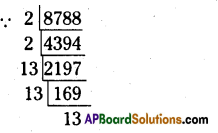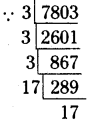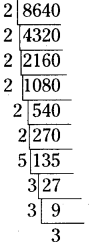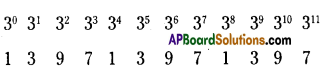AP State Syllabus AP Board 8th Class Maths Solutions Chapter 6 Square Roots and Cube Roots Ex 6.4 Textbook Questions and Answers.

## AP State Syllabus 8th Class Maths Solutions 6th Lesson Square Roots and Cube Roots Exercise 6.4Question 1.
Find the cubes of the following numbers
(1) 8
(ii) 16
(iii) 21
(iv) 30
Solution:

 Number Cube Of a Number i) 8 83 =  8 × 8 × 8 = 512 ii) 16 163 = 16 × 16 × 16 = 4096 iii) 21 213 = 21 × 21 × 21 = 9261 iv) 30 303 = 30 × 30 × 30 = 27000

Question 2.
Test whether the given numbers are perfect cubes or not.
(i) 243
(ii) 516
(iii) 729
(iv) 8000
(v)2700
Solution:

 Number Cube Of a Number Yes / No i) 243 3 × 3 × 3 × 3 × 3 = 35 No ii) 516 2 × 2 × 3 × 43 No iii) 729 9 × 9 × 9 = 93 Yes iv) 8000 20 × 20 × 20 = (20)3 Yes v) 2700 (30) × (30) × 3 NoQuestion 3.
Find the smallest number by which 8788 must be multiplied to obtain a perfect cube?
Solution:
The prime factorisation of 8788
= (2 × 2) × (13 × 13 × 13)∴ From the above product 2 ¡s left in the triplet.
∴ 2 should be multiplied with 8788 we will get a perfect cube number.

Question 4.
What smallest number should 7803 be multiplied with so that the product becomes a perfect cube?
Solution:
The prime factorisation of 7803
= (3 × 3 × 3) × (17 × 17)∴ From the above product 17 is left in
the triplet.
∴ 17 should be multiplied to 7803 then we will get a perfect cube number.Question 5.
Find the smallest number by which 8640 must be divided so that the quotient is a perfect cube’?
Solution:
The prime factorisation of 8640
= (2 × 2 × 2) × (2 × 2 × 2) × 5 × (3 × 3 × 3)
= (2)3 × (2)3 × 5 × (3)3Question 6.
Ravi made a cuboid of plasticine ofdimensions 12cm, 8cm and 3cm. How many minimum number of such cuboids will be needed to form a cube’?
Solution:
The volume of a plasticine cuboid
= l × b × h
= 12 × 8 × 3
= 288 cm3
If the minimum no. of such cuboids will be needed to form a cube then its volume be less than 288 i.e., 216 cm3
∴ s3 = 216
s = $$\sqrt{216}=\sqrt{6 \times 6 \times 6}=\sqrt{6^{3}}$$ = 6
∴ The side of the cube 6 cmQuestion 7.
Find the smallest prime number dividing the sum 311 +513.
Solution:
The units digit in 311 is 7∴ The units digit in 311 is 7
The units digit in 513 is 5
7 + 5 = 12 is divided by a smallest prime number 2.
∴ The smallest prime number that divide the sum 311 + 513 = 2